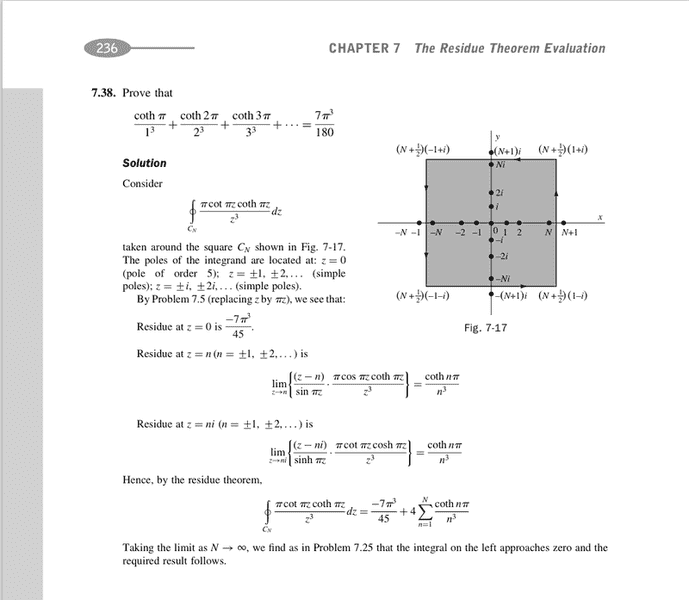# How to deal with this sum complex analysis?

## Homework StatementDown

## The Attempt at a Solution

As you see in the solution, I am confused as to why the sum of residues is required.

My question is the sum:

$$(4)\cdot\sum_{n=1}^{\infty} \frac{\coth(\pi n)}{n^3}$$

Question #1:

-Why is the beginning n=1 the residue is for all $$n \in (-\infty, \infty)$$ so the beginning of the sum should be $$n=-\infty$$ right?

Question #2:

Also in the residue theorem, it states:

$$\oint_{C} f(z) dz = (2\pi i)(\sum \text{Res})$$

Where is the 2pi(i) gone in the solution of theirs?

Thanks!

Dick
Homework Helper

## The Attempt at a Solution

As you see in the solution, I am confused as to why the sum of residues is required.

My question is the sum:

$$(4)\cdot\sum_{n=1}^{\infty} \frac{\coth(\pi n)}{n^3}$$

Question #1:

-Why is the beginning n=1 the residue is for all $$n \in (-\infty, \infty)$$ so the beginning of the sum should be $$n=-\infty$$ right?

Question #2:

Also in the residue theorem, it states:

$$\oint_{C} f(z) dz = (2\pi i)(\sum \text{Res})$$

Where is the 2pi(i) gone in the solution of theirs?

Thanks!

The limit of the contour integral is 0 as n->infinity. So the sum of all of the pole residues is 0. So ##2 \pi i## is a common factor of all of the residues. It cancels. The residue at 0 is the ##-7 \pi^3/45## part. For n>0 the residue is the same at n, -n, ni and -ni. So you can sum them all together by adding a factor of 4. The means you only have to sum over n>0.